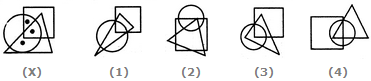# Non Verbal Reasoning - Dot Situation - Discussion

### Discussion :: Dot Situation - Section 1 (Q.No.9)

From amongst the figures marked (1), (2), (3) and (4), select the figure which satisfies the same conditions of placement of the dots as in figure (X).

9.

Select the figure which satisfies the same conditions of placement of the dots as in Figure-X.[A]. 1 [B]. 2 [C]. 3 [D]. 4

Explanation:

In fig. (X), one of the dots lies in the region common to the circle and the triangle only, another dot lies in the region common to all the three figures - the circle, the square and the triangle and the third dot lies in the region common to the circle and the square only. In each of the figures (1) and (3), there is no region common to the circle and the square only and in fig. (2), there is no region common to the circle and the triangle only. Only fig. (4) consists of all the three types of regions.

 Rushi Nichit said: (Jun 4, 2020) It should be an option no 3. Because there is square common.

 Teju said: (Oct 8, 2020) The Answer should be 2.